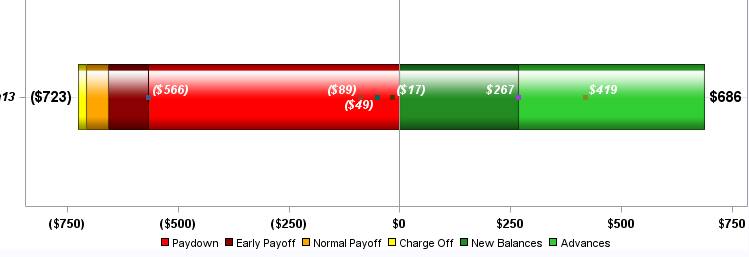## How to reorder stacked bar subgroups

My version is SAS 9.3. I am trying to figure out how to reorder the subgroups of this barchart. I am using legend overlaying a scatterplot with markers. The red segment needs to be moved to the furthest left with dark red, orange and yellow (up against the zero axis). My code is below the image.``````ods graphics / reset width=8in height=3in imagename='Wfall_GTL';
proc template;

define statgraph StackedClusterBarStat;
begingraph /
designheight = 750 designwidth = 1000 border = false
backgroundcolor=white drawspace=datavalue
entrytitle textattrs=(color=black style=italic size=16pt) "Example";
value "Paydown" / fillattrs=(color=red);
value "EPayoff" / fillattrs=(color=darkred);
value "NPayoff" / fillattrs=(color=orange);
value "COff" / fillattrs=(color=yellow);
value "New" / fillattrs=(color=forestgreen);

enddiscreteattrmap;
discreteattrvar attrvar=categoryfill var=type attrmap='fillcolors';
discreteattrvar attrvar=categoryfill var=type attrmap='fillcolors';
legendItem type=fill name="A" /
fillattrs=(color=red) outlineattrs=(color=red)
label="A" ;
legendItem type=fill name="B" /
fillattrs=(color=darkred) outlineattrs=(color=darkred)
label="B" ;
legendItem type=fill name="C" /
fillattrs=(color=orange) outlineattrs=(color=orange)
label="C" ;

legendItem type=fill name="D" /
fillattrs=(color=yellow) outlineattrs=(color=yellow)
label="D" ;
legendItem type=fill name="E" /
fillattrs=(color=forestgreen) outlineattrs=(color=forestgreen)
label="E" ;
legendItem type=fill name="F" /
fillattrs=(color=limegreen) outlineattrs=(color=limegreen)
label="F" ;

layout overlay / xaxisopts=(display=(ticks tickvalues) tickvalueattrs=(size=12 color=black weight=bold))
yaxisopts=(griddisplay=on /*offsetmin=0 offsetmax=.1*/ display=(ticks tickvalues)
tickvalueattrs=(Color=black Size=12 Style=Italic Weight=Bold)
linearopts= (viewmax=900 viewmin=-900
tickvaluesequence=(start=-900 end=900 increment=200)));

barchart x=month y=balance2 / orient=horizontal group=categoryfill groupdisplay=stack
barwidth=.4 barlabel=true /*primary=true*/ yaxis=y
barlabelattrs=(size=14 weight=bold color=black) barlabelformat=dollarc.
scatterplot x=balance2 y=month / /*markercharacter=balance2
markercharacterattrs=(size=12 color=white weight=bold)*/
group=categoryfill yaxis=y groupdisplay=overlay grouporder=ascending
markerattrs=(symbol=squarefilled)
datalabel=balance2 datalabelposition=auto
DATALABELATTRS=(Color=white Size=12 Style=Italic Weight=Bold);
discretelegend "A" "B" "C" "D" "E" "F" / valueattrs=(size=12 color=black)
autoitemsize=true location=outside halign=center valign=bottom border=false;

endlayout;
endgraph;
end;
run;
/*--Render the graph--*/
proc sgrender data=temp_merge template=StackedClusterBarStat;
format balance2 dollarc.; run;

``````
1 ACCEPTED SOLUTION

Accepted Solutions

## Re: How to reorder stacked bar subgroups

Usually I sort the data in the order that I want the categories to appear, and then use GROUPORDER=DATA on the BARCHART statement (or any other statement that supports it).

3 REPLIES 3

## Re: How to reorder stacked bar subgroups

Usually I sort the data in the order that I want the categories to appear, and then use GROUPORDER=DATA on the BARCHART statement (or any other statement that supports it).

## Re: How to reorder stacked bar subgroups

Thank you. I sorted the data the way I want to see it in the code. I took your suggestion and added grouporder=data in the barchart. Grouporder=data is already in the scatterplot code. The markers (from scatterplot) did not shift. They remain the same as illustrated below. Essentially, I would like the markers to sync up with the segments of the bar as midpoints in the barchart subgroups.

## Re: How to reorder stacked bar subgroups

I made some adjustments and it works for the most part. Thank you

Discussion stats
• 3 replies
• 1036 views
• 0 likes
• 2 in conversation